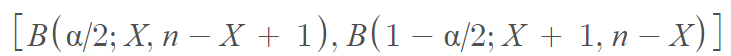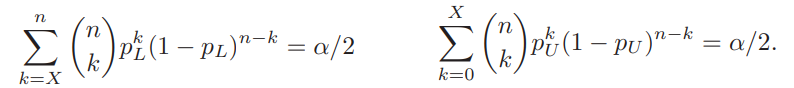# Clopper-Pearson Exact Method

Confidence Intervals > Clopper-Pearson

The Clopper-Pearson interval, also called the exact interval is an alternative to calculating binomial confidence intervals using normal approximation. It is based on inverting the equal-tailed binomial tests. It is the most commonly cited exact method for finding a confidence interval .

The Clopper-Pearson exact method is strictly conservative and therefore considered to be exact. As the method is conservative, it may not give the shortest possible interval containing the desired confidence interval . This is especially true when you know the population size. Although the Clopper-Pearson exact method was intended to have a minimum coverage of 95% , in the majority of cases the coverage can exceed 99%.

## Clopper-Pearson Exact Method Formula

The formula for the Clopper-Pearson exact method uses the relationship between the Binomial distribution and Beta distribution to form the interval.

The formula for the Clopper-Pearson interval is :where B(·; α, β) is the beta distribution quantile function with shape parameters α and β.

Given an observation , the lower P and upper limits are given by :## References

 Szekely, G. et al. [Eds.] (2000). Statistics for the 21st Century. Taylor & Francis.
 Helwig, N. (2020). Inference for proportions. Retrieved May 28, 2021 from: http://users.stat.umn.edu/~helwig/notes/ProportionTests.pdf
 Clopper,C.J.,and Pearson,E.S.(1934),“The Use of Confidence or Fiducial Limits Illustrated in the Case of the Binomial”, Biometrika 26, 404–413.
 Thulin, M. (2014). The cost of using exact confidence
intervals for a binomial proportion. Electronic Journal of Statistics. Vol 8. 817-840.

CITE THIS AS:
Stephanie Glen. "Clopper-Pearson Exact Method" From StatisticsHowTo.com: Elementary Statistics for the rest of us! https://www.statisticshowto.com/clopper-pearson-exact-method/